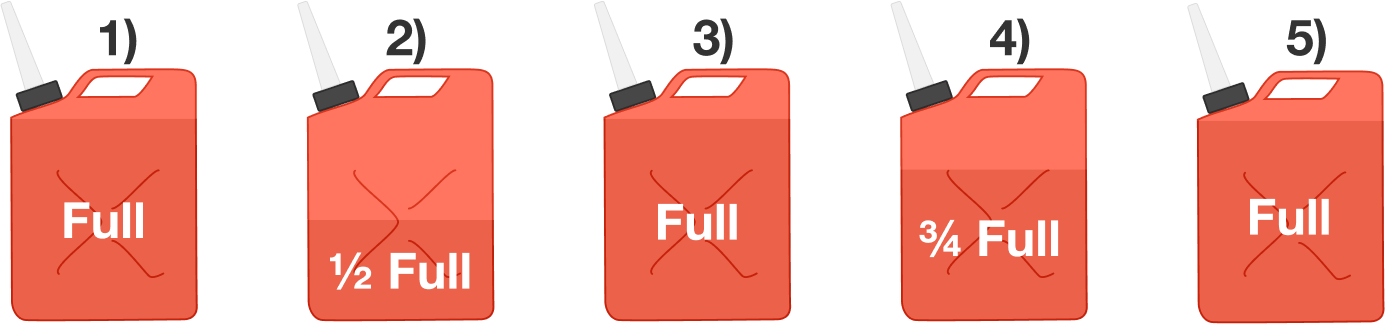# Gas Tank

Algebra Level 2

Consider the following sequence of events:

$\hspace{2mm}$ 1. Start with a full gasoline tank.
$\hspace{2mm}$ 2. Use half the gas.
$\hspace{2mm}$ 3. Re-fill the tank with gasoline until it's full.
$\hspace{2mm}$ 4. Use a quarter of the gas.
$\hspace{2mm}$ 5. Re-fill the tank with gasoline until it's full.

What percentage of the gas in the tank after step (5) was originally there in step (1)?

Note: Assume perfect mixing between steps. Enter your answer as a percentage. For example, 58.4% would be entered as 58.4.×